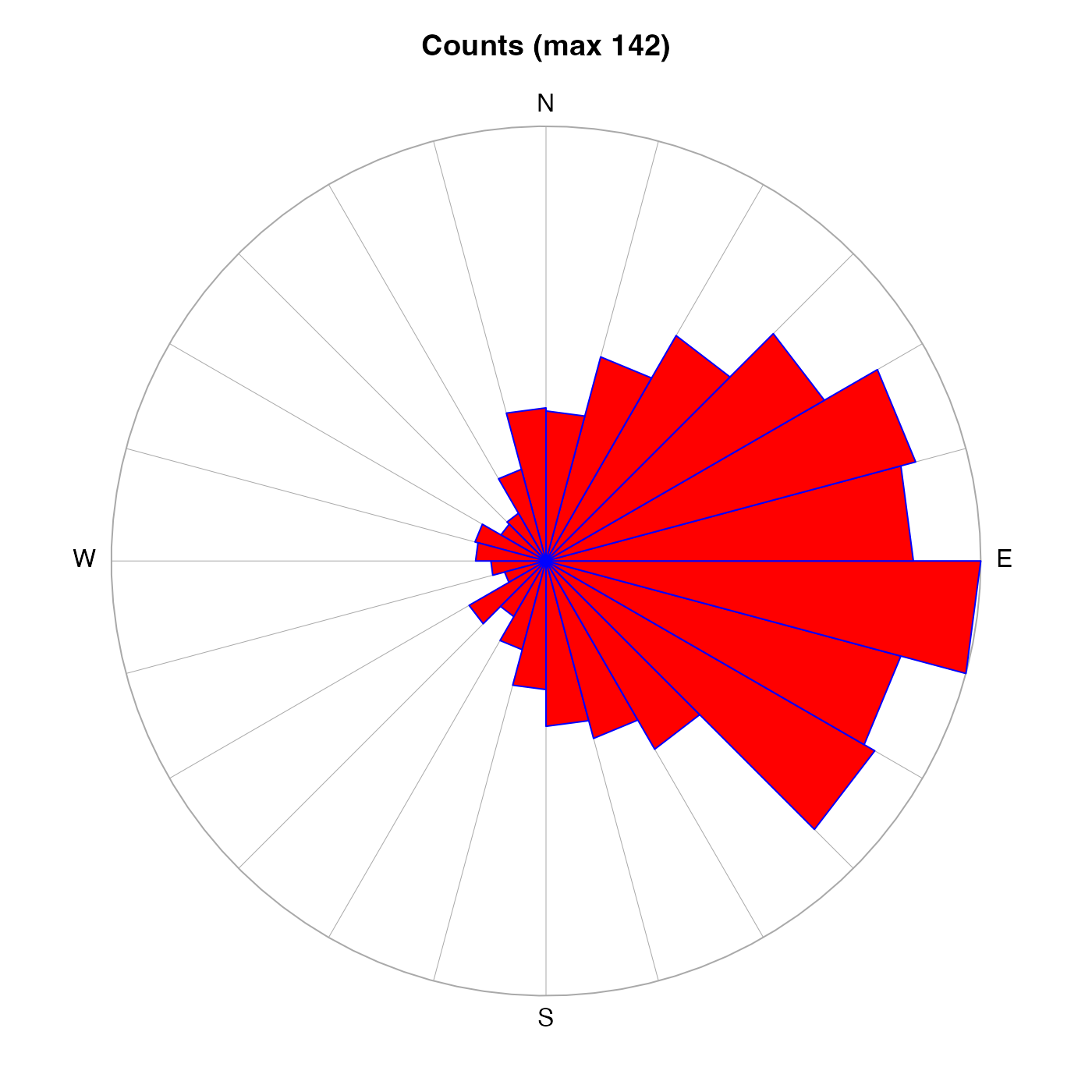Plot a windrose object.

# S4 method for windrose
plot(
x,
type = c("count", "mean", "median", "fivenum"),
convention = c("meteorological", "oceanographic"),
mgp = getOption("oceMgp"),
mar = c(mgp, mgp, 1 + mgp, mgp),
col,
...
)

## Arguments

x

a windrose object.

type

The thing to be plotted, either the number of counts in the angle interval, the mean of the values in the interval, the median of the values, or a fivenum() representation of the values.

convention

String indicating whether to use meteorological convention or oceanographic convention for the arrows that emanate from the centre of the rose. In meteorological convection, an arrow emanates towards the right on the diagram if the wind is from the east; in oceanographic convention, such an arrow indicates flow to the east.

mgp

Three-element numerical vector to use for par(mgp), and also for par(mar), computed from this. The default is tighter than the R default, in order to use more space for the data and less for the axes.

mar

Four-element numerical vector to be used with par("mar").

col

Optional list of colors to use. If not set, the colors will be c("red", "pink", "blue", "lightgray"). For the first three types of plot, the first color in this list is used to fill in the rose, the third is used for the petals of the rose, and the fourth is used for grid lines. For the "fivenum" type, the first color is used for the inter-quartile range, the second is used outside this range, the third is used for the median, and the fourth is, again, used for the grid lines.

...

Optional arguments passed to plotting functions.

Other functions that plot oce data: download.amsr(), plot,adp-method, plot,adv-method, plot,amsr-method, plot,argo-method, plot,bremen-method, plot,cm-method, plot,coastline-method, plot,ctd-method, plot,gps-method, plot,ladp-method, plot,landsat-method, plot,lisst-method, plot,lobo-method, plot,met-method, plot,odf-method, plot,rsk-method, plot,satellite-method, plot,sealevel-method, plot,section-method, plot,tidem-method, plot,topo-method, plot,xbt-method, plotProfile(), plotScan(), plotTS(), tidem-class

Other things related to windrose data: [[,windrose-method, [[<-,windrose-method, as.windrose(), summary,windrose-method, windrose-class

Dan Kelley

## Examples

library(oce)